# Impact of Loops and Memory on GP Fitness Landscapes

W. B. Langdon
Computer SciencePaper presented at EuroGP-2006 pages 225-237 details

# Introduction

• By adding memory and loops to linear GP we make it Turing complete, but
• What is effect of this on fitness landscape?
• T7 computer
• Experiments and results
• Mathematical models
• Implications

# GP is evolving O(1) functions

• Only a few papers published on evolving Turing complete programs.
• Foundations of Genetic Programming shows distribution of GP programs tends to a limit as programs gets bigger• Also true for Turing complete programs?
• In limit, fraction of halting T7 programs is zero

# T7 Minimal Turing Complete CPU

• 7 instructions
• Arithmetic unit is ADD. From + all other operations can be obtained. E.g.
• Boolean logic
• Multiply, by repeated addition (subroutines)
• Conditional (Branch if oVerflow flag Set)
• Move data in memory
• Save and restore Program Counter (i.e. Jump)
• Stop if reach end of program

# T7 Architecture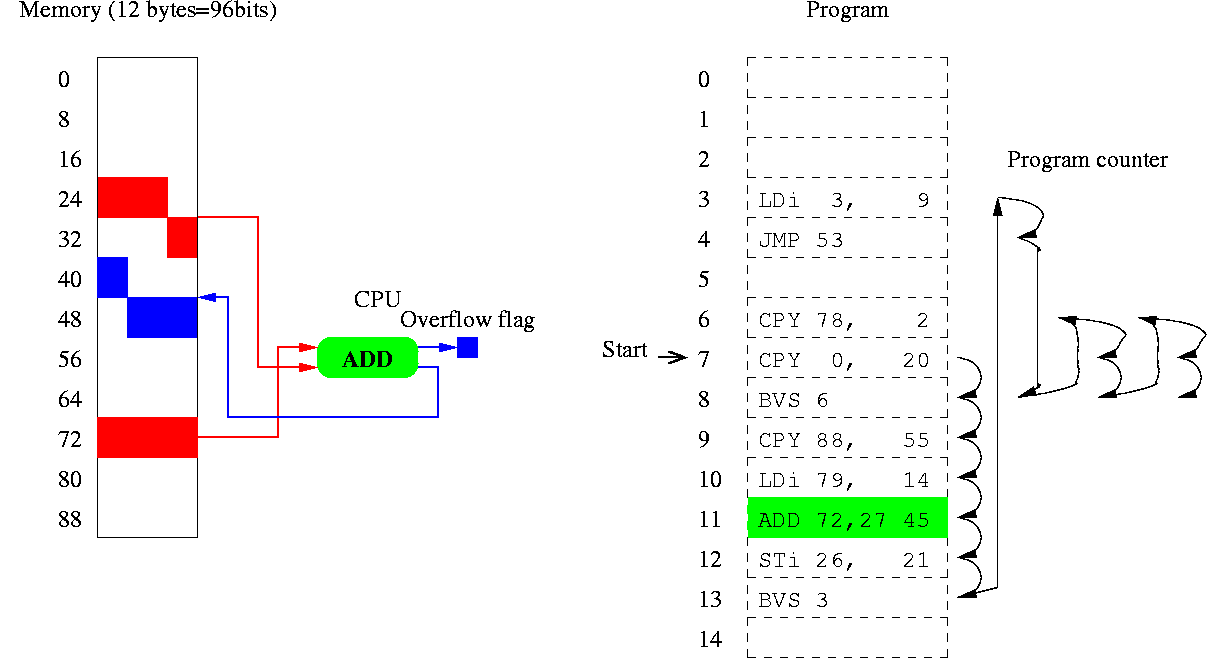# Experiments

• There are too many programs to test them all. Instead we gather statistics on random samples.
• Chose set of program lengths 30 to 16777215 (longest GP ever)
• Generate 1000 programs of each length
• Run them from random start point with random input
• Program terminates if it obeys the last instruction (which must not be a jump)
• How many stop?

# Controlling Run Away Programs

• Since our machine is finite, in principle it is possible to detect every infinite loop. But large memory and elapse time may be needed.
• Heuristic. Abort program if :
• any instruction is executed more >100 times.
• on re-executing any instruction, computer's state is identical to state when it was last executed.

# Almost all T7 Programs Loop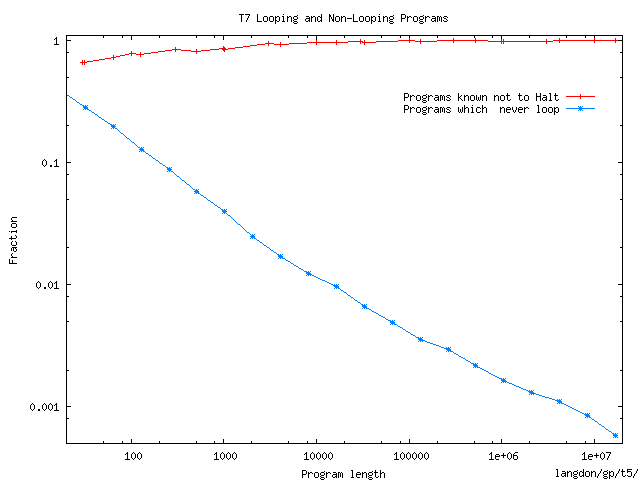# Model of Random Programs

• Before any repeated instructions;
• random sequence of instructions and
• random contents of memory.
• 1 in 7 instructions is a jump to a random location
• T7 instruction set chosen to have little bias.
• I.e. every state is ≈equally likely.
• Overflow flag set half the time.
• So 50% of conditional jumps BVS are active.
• (1+0.5)/7 instructions takes program counter to a random location.
• Implies for long programs, lengths of continuous instructions (i.e. without jumps) follows a geometric distribution with mean 7/1.5=4.67

# A Program Segment - Random code ending with a random jump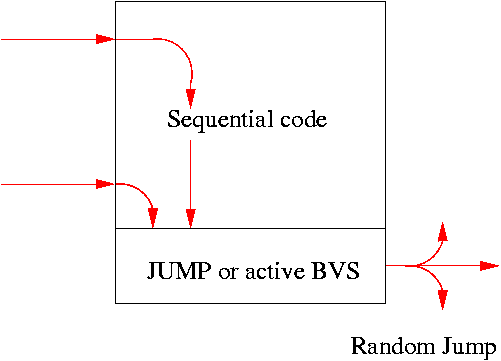# Forming Loops:Segments model

• Segments model assumes whole program is broken into N=L/4.67 segments of equal length of continuous instructions.
• Last instruction of each is a random jump.
• By the end of each segment, memory is re-randomised.
• Jump to any part of a segment, part of which has already been run, will form a loop.
• Jump to any part of the last segment will halt the program.
• Detailed and more accurate model in technical report CSM-445

# Probability of Halting

• i segments run so far. Chance next segment will
• Form first loop = i/N
• Halt program = 1/N.
• (so 1-(i+1)/N continues)
• Chance of halting immediately after segment i
• = 1/N × (1-2/N) (1-3/N) (1-4/N) … (1-i/N)
• = N-i (N-2)! /(N-i-1)!
• = (N-2)! N(1-N) N(N-1-i) /(N-i-1)!
• = (N-2)! N(1-N) eN Poisson(N-i-1,N)
• Total halting probability given by adding these gives ≈ sqrt(π/(2N)) = O(N)

# Proportion of Programs without loops falls as 1/sqrt(length)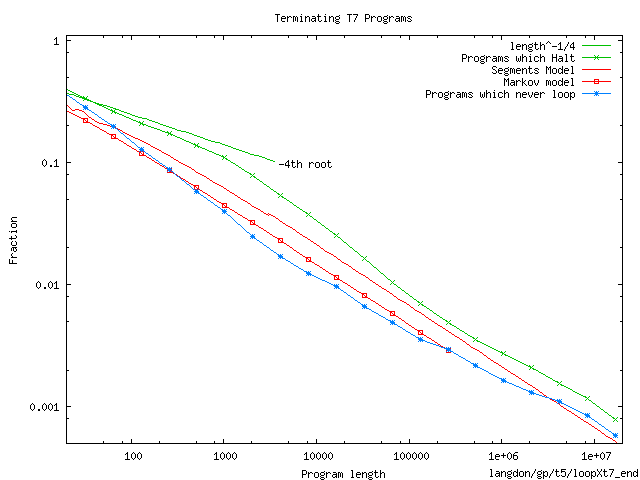Segments model over, but gives 1/√x scaling.
Approx 1% not proved to loop forever

# Number of Halting Programs rises exponentially with length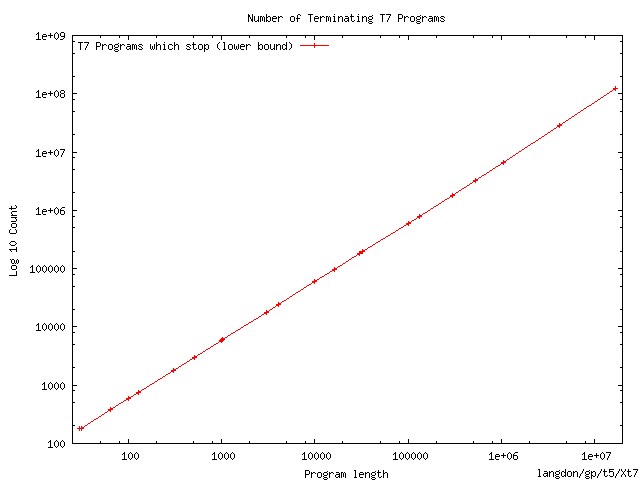The number of halting T7 programs containing 16 million instructions exceeds 10100 000 000.

# Average Run Time (non-looping)

• Mean run time given by
• Sum i weighting for segment i
∑ i ×(N-2)!N(1-N)eN Poisson(N-i-1,N)
Divided by sum of weightings (i.e. without × i)
∑ (N-2)!N(1-N)eN Poisson(N-i-1,N)
• We can bound upper part of fraction. (For long program its near one)
• Lower part gives sqrt(1/N)
• So expected run time grows as O(N½)

# Run time on Terminating Programs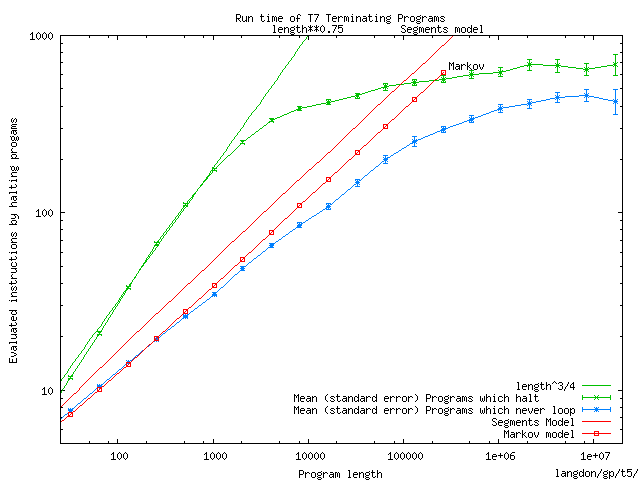Run time of non-looping programs fits Markov prediction.
Mean run time of all terminating programs length¾
Assuming maximum run time is limited by small, 12 bytes, memory becoming non-random.

# Suppose we have a GHz T7 computer

• In 1 millisecond it executes a million instructions
• If run time of halting programs follows a geometric distribution, 99.999% of halting programs halt within 11.5×average halting time.
• Empirically average halting time = length0.75
• 99.999% of halting programs with a million instructions will execute less than 12×(106)¾ ≈ 12×3.16×104 ≈ 360000 instructions.
• I.e. 99.999% terminate in < 0.36mS or not at all.

# Conclusions 1

• The halting probability falls with length to 0
• Number of terminating T7 programs rises exponentially with program size.
• Models explain inverse square root scaling
• Run time of halting programs rises slowly.
• So can be confident a randomly chosen program is looping if it does not stop almost immediately.

# The answer to the halting problem is No

• Number of T7 programs grows with size.
• So almost all programs are infinitely long.
• Infinitely long program are very unlikely to stop.
• With probability one, programs do not halt.